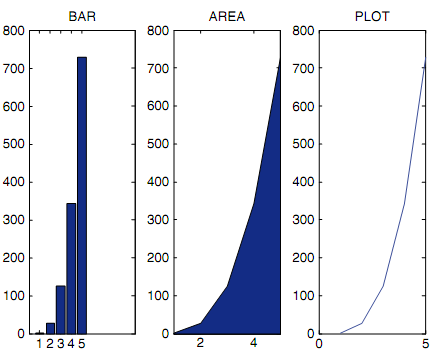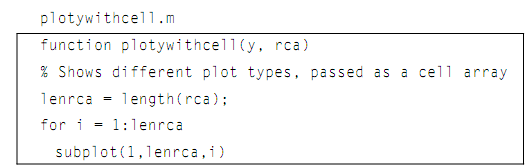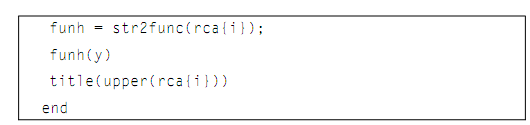## Plotting from a function, MATLAB in Engineering

Assignment Help:

Plotting from a Function:

The following function creates a Figure Window as shown in figure, which shows various types of plots for similar y vector. The vector is passed as an input argument to the function, as in a cell array with the plot type names. The function creates the Figure Window by using the cell array with the plot type names. It generates a function handle for each using the str2func function.#### Vector operations, Vector operations: As vectors are special cases of ...

Vector operations: As vectors are special cases of matrices, the matrix operations elaborated (addition, subtraction, multiplication, scalar multiplication, transpose) work on

#### Basic mathematical operations, Basic mathematical operations: All the ...

Basic mathematical operations: All the basic mathematical operations can be executed on symbolic expressions and variables (example, add, raise to a power, multiply, subtract,

#### Sound files, Sound Files: The sound signal is an illustration of a con...

Sound Files: The sound signal is an illustration of a continuous signal which is sampled to result in a discrete signal. In this situation, sound waves traveling through the a

#### Gauss-jordan, Gauss-Jordan: The Gauss-Jordan elimination technique beg...

Gauss-Jordan: The Gauss-Jordan elimination technique begins in similar way which the Gauss elimination technique does, but then rather than of back-substitution, the eliminati

#### Signals, Q.1: Consider the transmission of a sinusoid x(t) = cos(2f0t) thr...

Q.1: Consider the transmission of a sinusoid x(t) = cos(2f0t) through a channel a ected by multipath and Doppler. Let there be two paths, and assume the sinusoid is being sent fro

#### Plotting from a function, Plotting from a Function: The following func...

Plotting from a Function: The following function creates a Figure Window as shown in figure, which shows various types of plots for similar y vector. The vector is passed as a

#### Inverse of square matrix, Inverse of square matrix: The inverse is, he...

Inverse of square matrix: The inverse is, hence the result of multiplying the scalar 1/D by each and every element in the preceding matrix. Note that this is not the matrix A,

#### Str2num function - string, str2num function - String: The function str2...

str2num function - String: The function str2num does the opposite; it takes the string in which a number is stored and converts it to the type double: >> num = str2num('123.

#### Variable scope, Variable Scope: The scope of any of variable is the wo...

Variable Scope: The scope of any of variable is the workspace in which it is valid. The workspace generated in the Command Window is known as the base workspace. As we know

#### Calcrectarea subfunction, calcrectarea subfunction: function call: ar...

calcrectarea subfunction: function call: area = calcrectarea(len,wid); function header: function area = calcrectarea(len, wid)   In the function call, the two arg